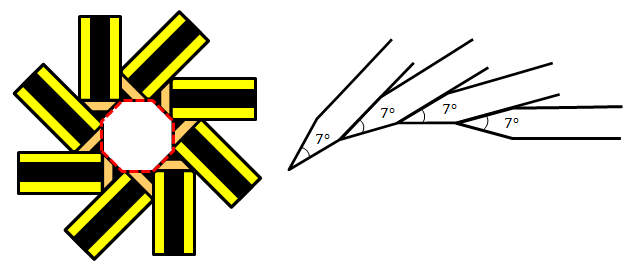# It All Came From Pencils 3We can make "pencilogons" by aligning multiple, identical pencils end-of-tip to start-of-tip together without leaving any gaps, as shown above, so that the enclosed area forms a regular polygon (the example above left is an 8-pencilogon).

Hazri wants to make an $n$-pencilogon using $n$ identical pencils with pencil tips of angle $7^\circ.$ After he aligns $n-18$ pencils, he finds out the gap between the two ends is too small to fit in another pencil.

So, in order to complete the pencilogon, he has to sharpen all the $n$ pencils so that the angle of all the pencil tips becomes $(7-m)^\circ$.

Find the value of $m+n$.

(Assume the pencils have a rectangular body and have their tips resembling isosceles triangles)

×